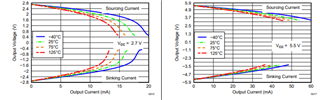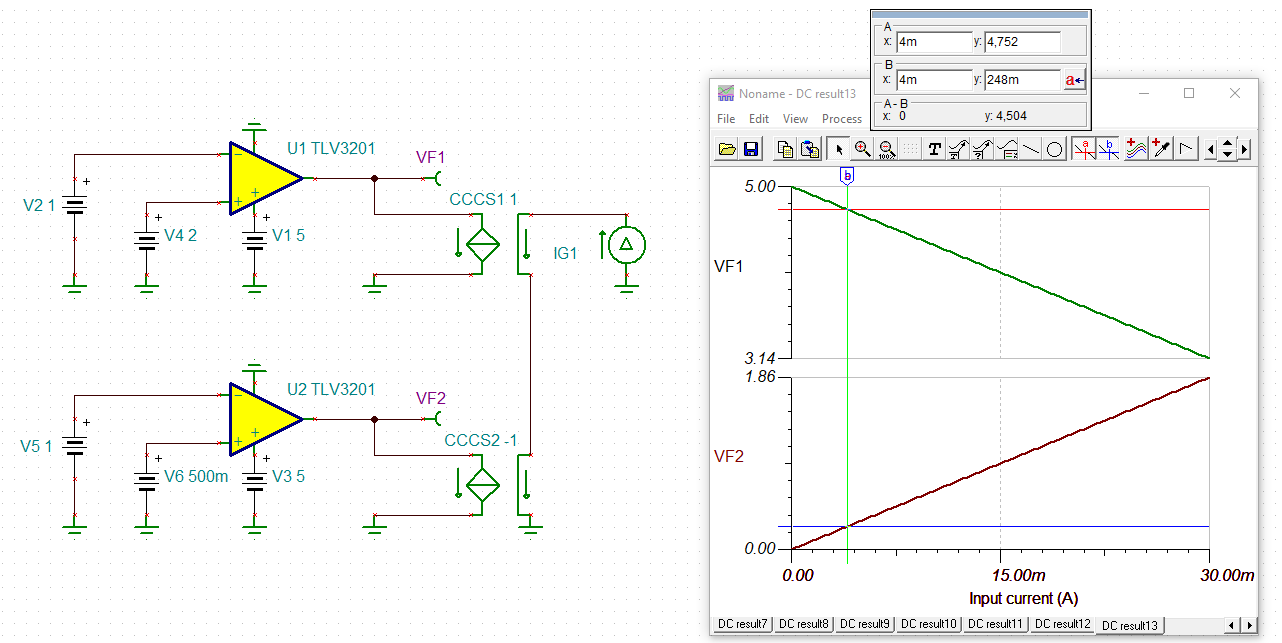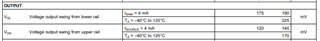This thread has been locked.

If you have a related question, please click the "Ask a related question" button in the top right corner. The newly created question will be automatically linked to this question.

# TLV3201-Q1: VOH, VOL calculation at any temperature

Part Number: TLV3201-Q1
Other Parts Discussed in Thread: TINA-TI, TLV3201

Hi Team,

Can you help explain how to calculate the VOH and VOL of this device at any temperature? Can you help me with these? Is there an application note that I can check?

I searched and found the documents, Amplifier Input Common-Mode and Output-Swing Limitations and Understanding Operational Amplifier Specifications but It is still unclear to me after reading.

Thank you.

Regards,

Marvin

• Marvin

Thanks for your post.  Unfortunately there is not a specific formula that you can use.  We recommend you work from these plots to estimate VOH and VOL at your specific temperature and operating supply.  VOH and VOL are worse at lower operating voltages.  To estimate the output impedance at a given output level and temperature, divide the voltage drop by the amount of current being sourced or sunk.  This value is fairly constant at a given temp and operating supply.

Chuck• Hi Chuck,

Thank you. Could you tell me the architecture? If calculating the VOH by the picture, Is it Vout = Vcc - VOH?, VOH = Iout * R?

Thank you.

Regards,

Marvin

• Hi Marvin,

running a TINA-TI simulation helps to find out what's going on, even if the shown output voltage differ a bit from the datasheet values:marvin_tlv3201.TSC

The TLV3201 is a CMOS comparator. So in a first order approximation the output voltage drop due the output current is simply caused by the turn-on resistance of according output MOSFET.

Of course, this is only valid for small output currents as can be seen by the linear shape of the curve. At higher output currents the voltage drop increases nonlinearily.

And, of course, the turn-on resistance varries with temperature, just like with every normal MOSFET.

Kai

• Thanks Kai for the excellent explanation

Chuck

• Hi Kai and Chuck,

Thank you for the explanation. The customer tried the simulation has the following question:

1. is the simple formula of Voh and Vol is Ron*Iout ?

The Voh in datasheet at -40~125 is 170mV(Vcc=5). does it means it may be 170mV at any temperature ?

Regards,

Marvin

• Marvin

thanks for reaching out again for clarification.

RON x Iout is the voltage drop or non ideal swing from the rail as shown in the datasheet.

The 170mV value for VOL is the worst case value at Vcc = 5V across the temp range.  As I showed earlier in the plots, it does vary across temp.   So no, it is not the same at all temps.

Chuck

.